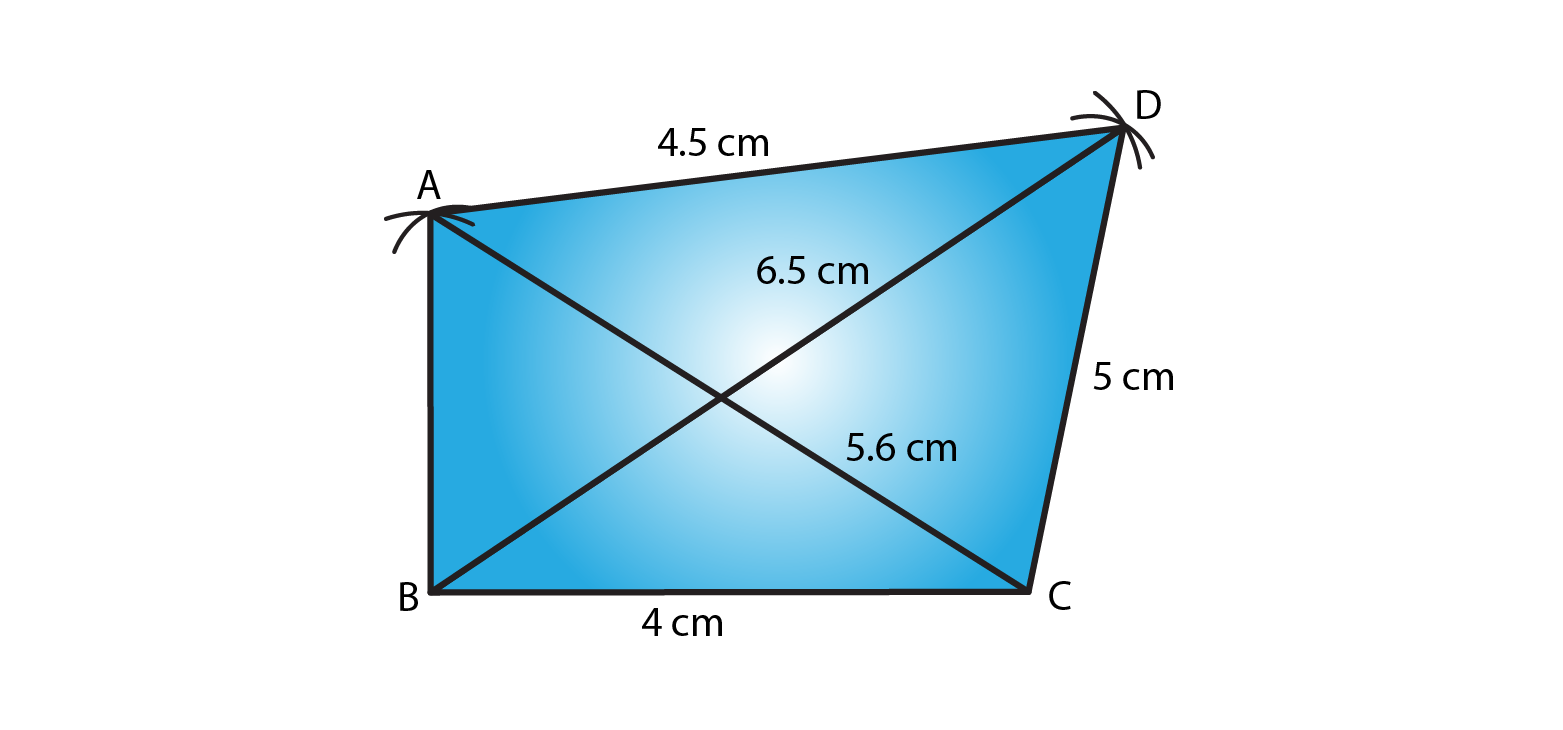# RD Sharma Solutions for Class 8 Maths Chapter 18 Practical Geometry (Constructions) Exercise 18.2

Exercise 18.2 mainly deals with concepts related to constructing a quadrilateral when its three sides and two diagonals are given. Students can self analyze the knowledge gained about the chapter by solving the solutions which are formulated by our expert tutors. Students aspiring to score high marks are advised to download and practice the solutions on a daily basis. RD Sharma Class 8 Solutions pdf are available here where students can download from the links provided below.

## Download the Pdf of RD Sharma Solutions for Class 8 Maths Exercise 18.2 Chapter 18 Practical Geometry (Constructions)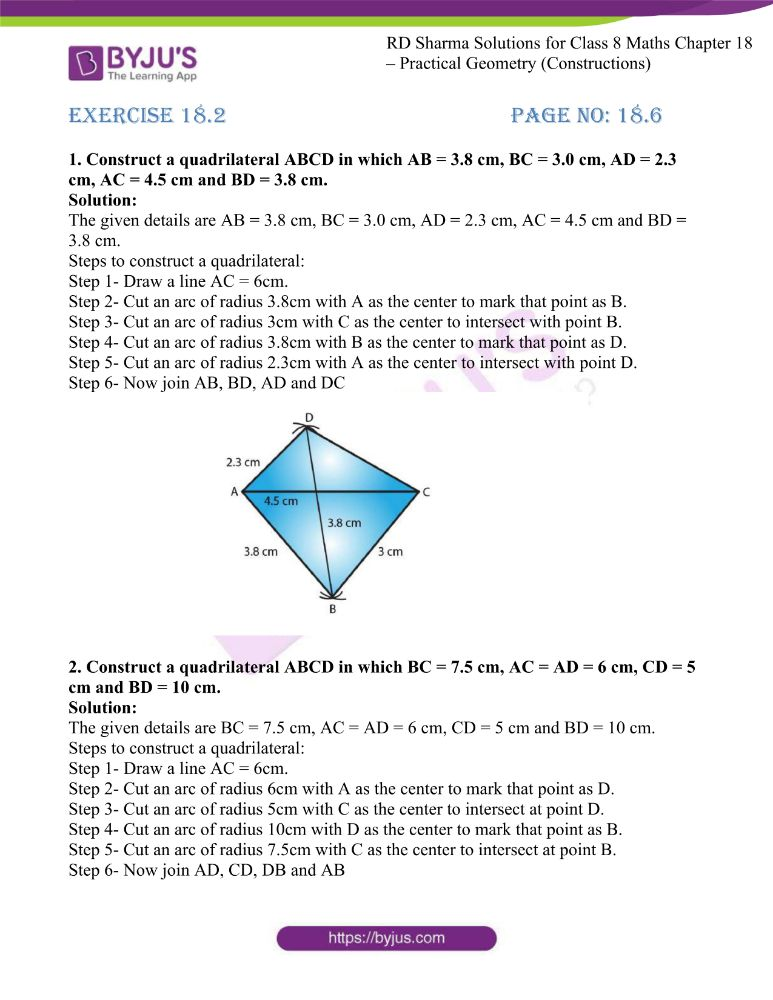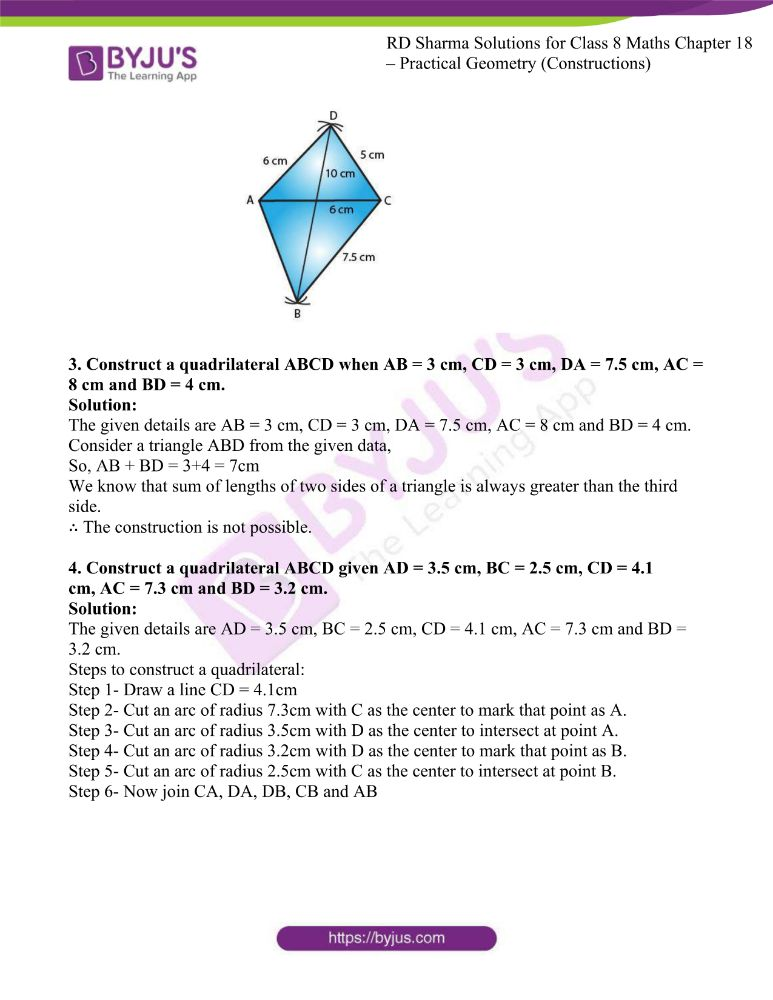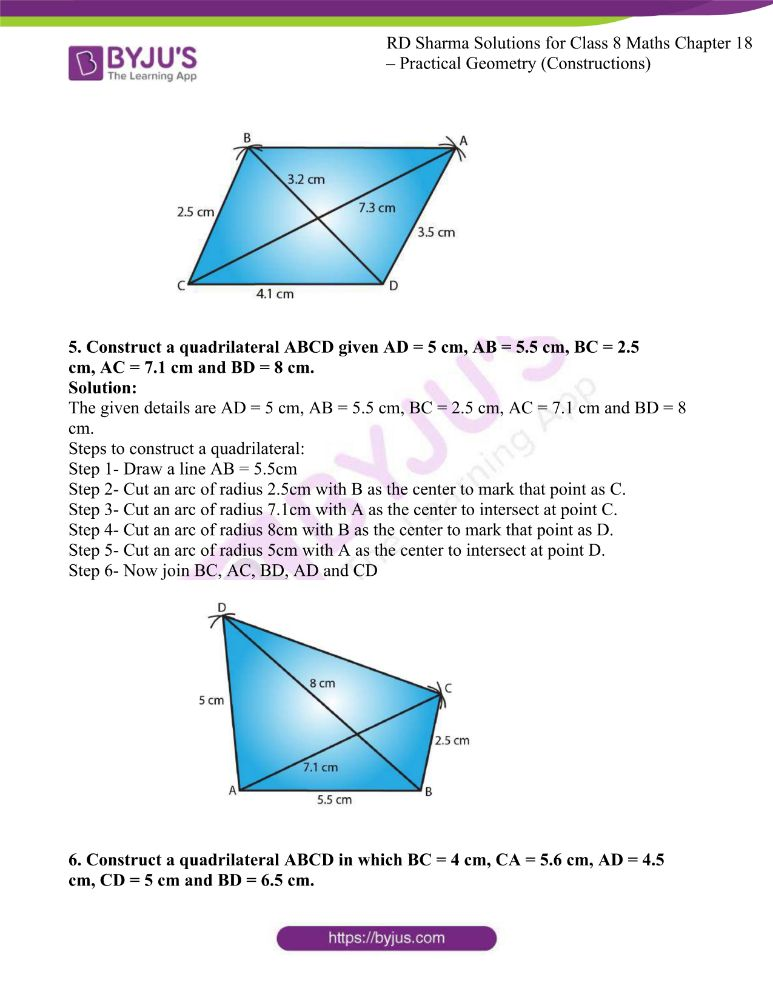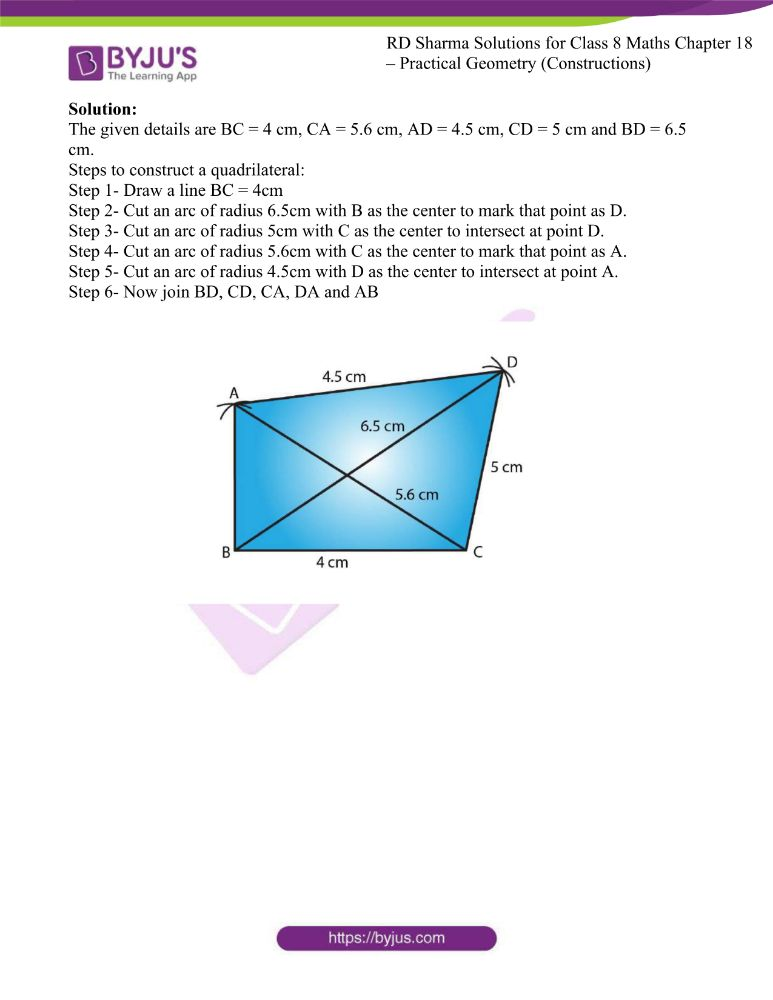### Access answers to RD Sharma Maths Solutions For Class 8 Exercise 18.2 Chapter 18 Practical Geometry (Constructions)

1. Construct a quadrilateral ABCD in which AB = 3.8 cm, BC = 3.0 cm, AD = 2.3 cm, AC = 4.5 cm and BD = 3.8 cm.

Solution:

The given details are AB = 3.8 cm, BC = 3.0 cm, AD = 2.3 cm, AC = 4.5 cm and BD = 3.8 cm.

Step 1- Draw a line AC = 6cm.

Step 2- Cut an arc of radius 3.8cm with A as the center to mark that point as B.

Step 3- Cut an arc of radius 3cm with C as the center to intersect with point B.

Step 4- Cut an arc of radius 3.8cm with B as the center to mark that point as D.

Step 5- Cut an arc of radius 2.3cm with A as the center to intersect with point D.

Step 6- Now join AB, BD, AD and DC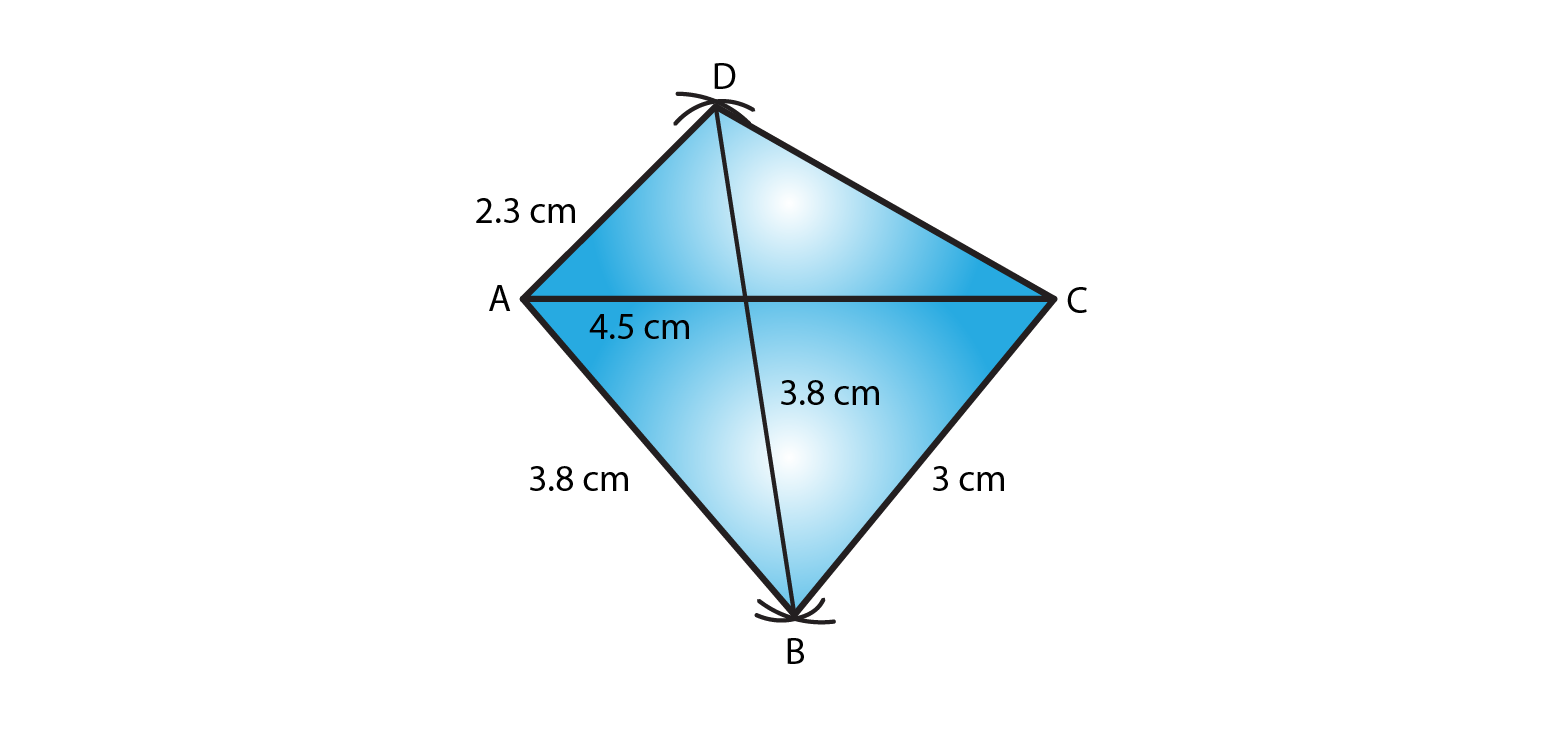2. Construct a quadrilateral ABCD in which BC = 7.5 cm, AC = AD = 6 cm, CD = 5 cm and BD = 10 cm.

Solution:

The given details are BC = 7.5 cm, AC = AD = 6 cm, CD = 5 cm and BD = 10 cm.

Step 1- Draw a line AC = 6cm.

Step 2- Cut an arc of radius 6cm with A as the center to mark that point as D.

Step 3- Cut an arc of radius 5cm with C as the center to intersect at point D.

Step 4- Cut an arc of radius 10cm with D as the center to mark that point as B.

Step 5- Cut an arc of radius 7.5cm with C as the center to intersect at point B.

Step 6- Now join AD, CD, DB and AB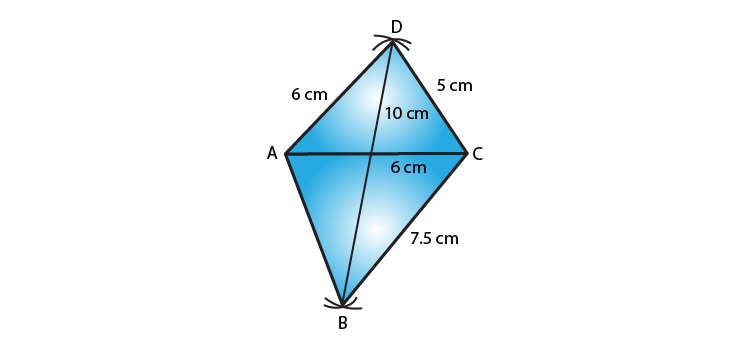3. Construct a quadrilateral ABCD when AB = 3 cm, CD = 3 cm, DA = 7.5 cm, AC = 8 cm and BD = 4 cm.

Solution:

The given details are AB = 3 cm, CD = 3 cm, DA = 7.5 cm, AC = 8 cm and BD = 4 cm.

Consider a triangle ABD from the given data,

So, AB + BD = 3+4 = 7cm

We know that sum of lengths of two sides of a triangle is always greater than the third side.

∴ The construction is not possible.

4. Construct a quadrilateral ABCD given AD = 3.5 cm, BC = 2.5 cm, CD = 4.1 cm, AC = 7.3 cm and BD = 3.2 cm.

Solution:

The given details are AD = 3.5 cm, BC = 2.5 cm, CD = 4.1 cm, AC = 7.3 cm and BD = 3.2 cm.

Step 1- Draw a line CD = 4.1cm

Step 2- Cut an arc of radius 7.3cm with C as the center to mark that point as A.

Step 3- Cut an arc of radius 3.5cm with D as the center to intersect at point A.

Step 4- Cut an arc of radius 3.2cm with D as the center to mark that point as B.

Step 5- Cut an arc of radius 2.5cm with C as the center to intersect at point B.

Step 6- Now join CA, DA, DB, CB and AB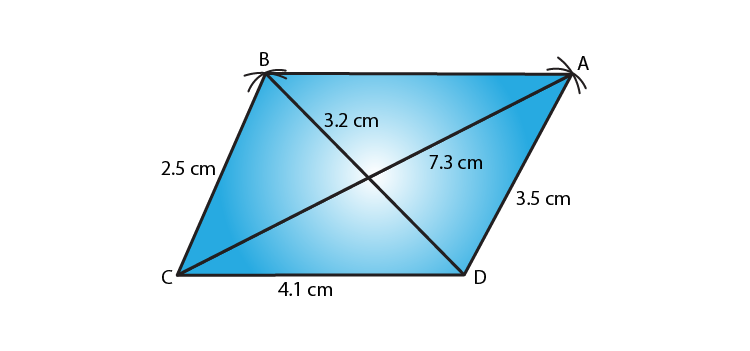5. Construct a quadrilateral ABCD given AD = 5 cm, AB = 5.5 cm, BC = 2.5 cm, AC = 7.1 cm and BD = 8 cm.

Solution:

The given details are AD = 5 cm, AB = 5.5 cm, BC = 2.5 cm, AC = 7.1 cm and BD = 8 cm.

Step 1- Draw a line AB = 5.5cm

Step 2- Cut an arc of radius 2.5cm with B as the center to mark that point as C.

Step 3- Cut an arc of radius 7.1cm with A as the center to intersect at point C.

Step 4- Cut an arc of radius 8cm with B as the center to mark that point as D.

Step 5- Cut an arc of radius 5cm with A as the center to intersect at point D.

Step 6- Now join BC, AC, BD, AD and CD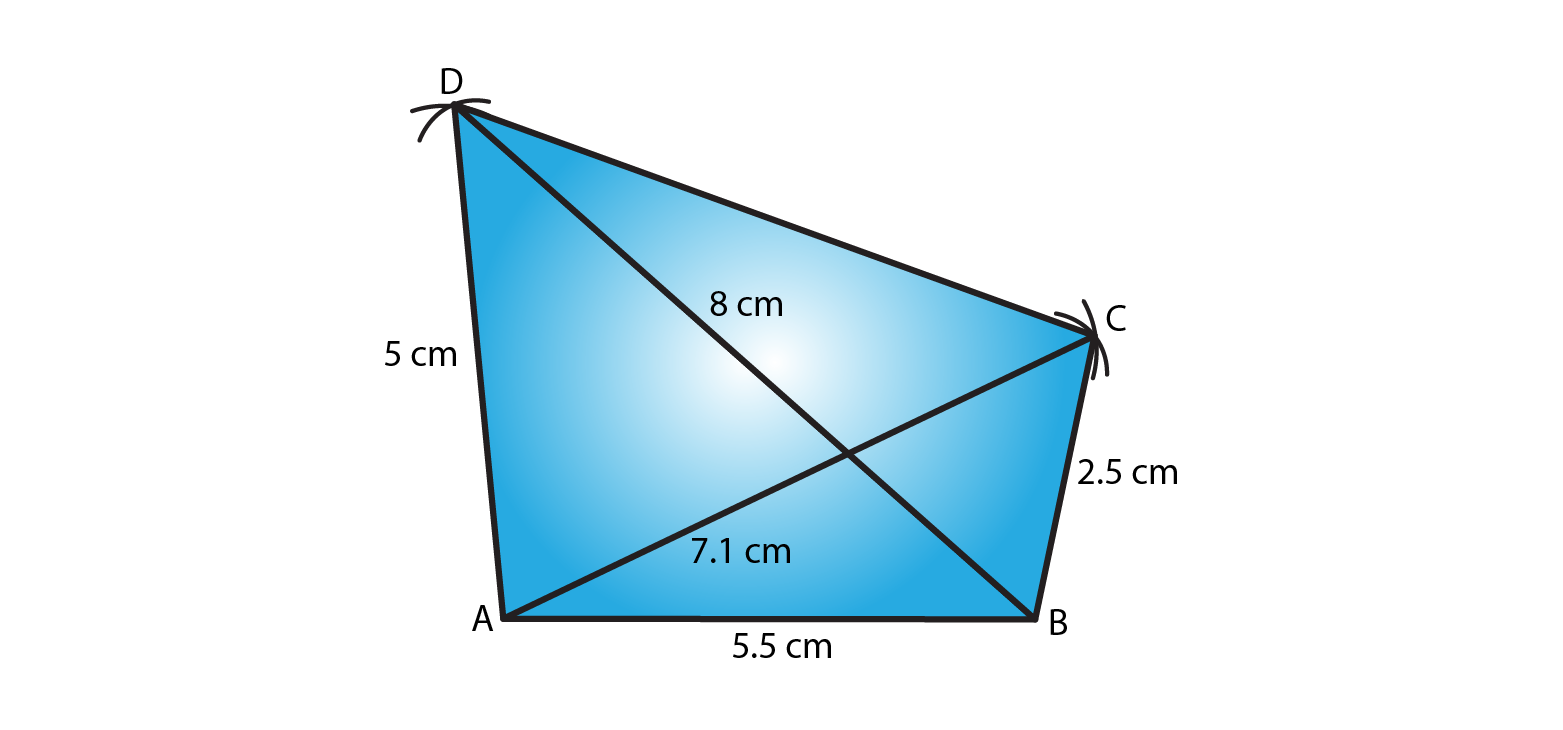6. Construct a quadrilateral ABCD in which BC = 4 cm, CA = 5.6 cm, AD = 4.5 cm, CD = 5 cm and BD = 6.5 cm.

Solution:

The given details are BC = 4 cm, CA = 5.6 cm, AD = 4.5 cm, CD = 5 cm and BD = 6.5 cm.

Step 1- Draw a line BC = 4cm

Step 2- Cut an arc of radius 6.5cm with B as the center to mark that point as D.

Step 3- Cut an arc of radius 5cm with C as the center to intersect at point D.

Step 4- Cut an arc of radius 5.6cm with C as the center to mark that point as A.

Step 5- Cut an arc of radius 4.5cm with D as the center to intersect at point A.

Step 6- Now join BD, CD, CA, DA and AB